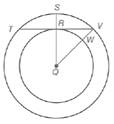Chapter 6.1, Problem 16EElementary Geometry For College St...

7th Edition
Alexander + 2 others
ISBN: 9781337614085

Solutions

Chapter
SectionElementary Geometry For College St...

7th Edition
Alexander + 2 others
ISBN: 9781337614085
Textbook Problem

Given: Concentric circles with center Q S R = 3  and  R Q = 4 Q S ¯ ⊥ T V ¯  at  R Find: a) R V b) T VExercises 16, 17

To determine

a)

To find: RV¯.

Explanation

Given:

Concentric circles with centre Q with SR=3,RQ=4 and QS¯ is perpendicular to TV¯ at R.

Definition:

If X is a point on AB¯ and A-X-B, then AX¯+XB¯=AB¯.

Calculation:

Given that QS¯ is perpendicular to TV¯.

Therefore QRV=90.

Hence ΔQRV is a right triangle.

By segment addition postulate, QS¯=SR¯+RQ¯.

We have SR=3,RQ=4

QS¯=SR¯+RQ¯=3+4QS¯=7

From the figure, we see that QS and QV are radii of the outer circle

To determine

b)

To find: TV¯.

Still sussing out bartleby?

Check out a sample textbook solution.

See a sample solution

The Solution to Your Study Problems

Bartleby provides explanations to thousands of textbook problems written by our experts, many with advanced degrees!

Get Started

Find the value of k such that (k,k) is equidistant from (1,0) and (0,2).

Finite Mathematics and Applied Calculus (MindTap Course List)

Evaluate the integrals in Problems 1-32. Identify the formula used. 11.

Mathematical Applications for the Management, Life, and Social Sciences

True or False: is a rational function.

Study Guide for Stewart's Single Variable Calculus: Early Transcendentals, 8th

Find for y defined implicity by .

Study Guide for Stewart's Multivariable Calculus, 8th